RUSSIAN JOURNAL OF EARTH SCIENCES, VOL. 16, ES5001, doi:10.2205/2016ES000577, 2016

# Threshold sensitivity of quartz torsion magnetometers on a base of vicalloy and samarium-cobalt

V. I. Odintsov, V. G. Petrov

N. V. Pushkov Institute of Terrestrial Magnetism, Ionosphere and Radio Wave Propagation, Russian Academy of Sciences, Moscow, Russia

### Abstract

Extremely achievable parameters of quartz torsion variometer magnetometers are investigated. Influence of instability and noise of the opto-electronic converter on a threshold of sensitivity of a magnetometer with the negative feedback (NF) is considered and extreme values of a sensitivity threshold of the magnetometer are estimated for different magnetic materials used, depths of NF and the demanded frequency range of measurements. Criteria for optimization of the sizes and a form of the magnets are determined by a condition of a maximum relation of the magnetic moment to the moment of inertia. Theoretical and experimental estimates of noise level of magnetometers on a base of vicalloy and samarium-cobalt are given. It is shown that for threshold sensitivity of the magnetometer about 1 pT, the frequency range of the magnetometer with a sensor on a base of vicalloy is limited by a frequency about 1.6 Hz, samarium-cobalt – 6.4 Hz. The received values of a frequency and threshold sensitivity for the specified magnetic materials completely meet the requirements of Intermaget for one-second data and are probably the extreme values for quartz variometers with the opto-electronic converter of angular movements.

### 1. Introduction

Due to the need of providing a geomagnetic observatories network of Russia with the magnetometers of a domestic manufacturing, which meet the requirements of the international Intermagnet network, and the planned transition of Intermagnet to one-second registration

(INTERMAGNET One second Data Standards. A Proposal for Comment, 2011 http://www-ssc.igpp.ucla.edu/gem/pdf/INTERMAGNET One-second Standards Proposal.pdf), extremely achievable parameters of magnetometers on the base of quartz torsion variometers [Bobrov, 1961; Yanovsky, 1963] are investigated. Use of the optical-electronic converter (OEC) in combination with the deep negative feedback (NF) [Bryunelli, 1959; Marianiuk, 1977] provided a wide circulation of magnetometers on the basis of quartz variometers in geomagnetic observatories [Askerov, 1989; Hegymegi, 1986; Jankowski, 1984; Odintsov, 1982]. Influence of instability and noise of OEC on a threshold of sensitivity of the magnetometer with NF is estimated and limit values of its threshold of sensitivity depending on a design of a variometer and the applied magnetic materials are received. It is shown that at threshold sensitivity of the magnetometer about 1 pT, the frequency range of the magnetometer with a variometer on a vicalloy is limited by a frequency of 1.6 Hz, samarium-cobalt – 6.4 Hz.

### 2. Main Equation of the Quartz Torsion Variometer

The quartz torsion variometer (quartz sensor QS) represents the moving permanent magnet with a small mirror hung on a quartz threads. Its dynamics is described by the differential equation of the second order [Yanovsky, 1963] to which there corresponds transfer function:

\begin{eqnarray*} W_1(p) = \frac{\theta(p)}{\delta B(p)} = \frac{M}{J_m p^2 + \xi p + W_M} \end{eqnarray*}

Dynamic characteristics of a variometer are defined completely by its angular natural frequency $\omega_{\mathrm{nat}}$ and damping factor $\beta_{\mathrm{nat}}$ to which there correspond expressions:

\begin{eqnarray*} \omega_{\mathrm{nat}}^2 = \frac{W_M}{J_m}, \qquad \beta_{\mathrm{nat}} = \frac{\xi}{2 \sqrt{W_M J_m}} \end{eqnarray*}

Taking into account these transfer function expressions will assume a form:

\begin{eqnarray*} W_1(p) = \frac{M}{W_M} \displaystyle{\frac{1} {(p/\omega_{\mathrm{nat}})^2 + 2 \beta_{\mathrm{nat}}(p/\omega_{\mathrm{nat}})+1}} \end{eqnarray*}

In the steady-state conditions, at $p=0$, $W_1(0)=M/W_M$, and product of square self-oscillation frequency of variometer on its conversion coefficient is constant and equal to the relation of the magnetic moment of a magnet by the mass moment of inertia of QS concerning an axis of its rotation:

\begin{eqnarray*} \omega_{\mathrm{nat}}^2 W_1(0) = \frac{M}{J_m} \end{eqnarray*}

In a manufacturing of quartz variometers the characteristic "scale value" $\varepsilon$, the inverse value to conversion coefficient of a variometer $W_1(0)$, is used. Relationship between $W_1(0)$ (rad/T) and $\varepsilon$ (nT m/mm) can be described as $W_1(0) = 5\times 10^5/\varepsilon$, then:

\begin{eqnarray*} \omega_{\mathrm{nat}}^2 W_1(0) = 1.267 \times 10^4 \;\frac{f_{\mathrm{nat}}^2}{\varepsilon} \end{eqnarray*}

The relation of a square of self-oscillation frequency of variometer to its scale value is known as "quality factor" of magnetic system of quartz variometer, and can serve as the quality assessment of a quartz variometer $(f_{\mathrm{nat}})^2/\varepsilon = 0.79 \times 10^{-4} M/J_m$.

### 3. Quartz Variometer With NF (The Quartz Magnetometer)

The quartz magnetometer [Odintsov, 1982] contains in addition to a variometer the optical-electronic converter of angular displacements of a magnet to an output current and a NF chain with transfer functions respectively:

\begin{eqnarray*} W_2(p) = K_{\mathrm{oec}}, \qquad W_3(p) = \frac{G_{\mathrm{nf}}}{R_{\mathrm{nf}}} \end{eqnarray*}

Transfer function of the magnetometer can be determined by the expression [Klyuev, 1973]:

\begin{eqnarray*} W_{\mathrm{nf}}(p) = \frac{W_1(p) W_2(p)}{1+ W_1(p) W_2(p) W_3(p)} = \end{eqnarray*} \begin{eqnarray*} \frac{R_{\mathrm{nf}}}{G_{\mathrm{nf}}} \: \frac{W_{\mathrm{EM}}}{J_mp^2 + \xi p + W_M + W_{\mathrm{EM}}} \end{eqnarray*}

As a result of NF action there is an additional "electromagnetic counteracting moment", which increases the variometer counteracting moment and which is equal: $W_{\mathrm{EM}} =M K_{\mathrm{oec}} G_{\mathrm{nf}}/R_{\mathrm{nf}}$. For an assessment of increase the concept "statizm" of a variometer with NF is used:

\begin{eqnarray*} \gamma_0 = \frac{W_M}{W_M + W_{\mathrm{EM}}} \end{eqnarray*}

Dynamic characteristics of the magnetometer – the self oscillation angular frequency and damping factor are connected with characteristics of a variometer through a statizm:

\begin{eqnarray*} \omega_0^2 = \frac{W_M + W_{\mathrm{EM}}}{J_m} = \frac{\omega_{\mathrm{nat}}^2}{\gamma_0} \end{eqnarray*} \begin{eqnarray*} \beta_0 = \frac{\xi}{2\sqrt{J_m(W_M + W_{\mathrm{EM}})}} = \beta_{\mathrm{nat}}\sqrt{\gamma_0} \end{eqnarray*}

By analogy with characteristics of a variometer itself, the expression for transfer function of the magnetometer in the presence of NF will assume a form:

\begin{eqnarray*} W_{\mathrm{nf}}(p) = \frac{R_{\mathrm{nf}}}{G_{\mathrm{nf}}} \: \frac{1-\gamma_0}{(p/\omega_0)^2 + 2 \beta_0 (p/\omega_0) +1} \end{eqnarray*}

From this expression follows that the conversion coefficient of the magnetometer in the steady-state conditions is equal

\begin{eqnarray*} W_{\mathrm{nf}}(0) = \frac{ (1- \gamma_0) R_{\mathrm{nf}}}{G_{\mathrm{nf}}} \end{eqnarray*}

and is defined with an error up to statizm $\gamma_0$ only by parameters of NF circuit and doesn't depend on parameters of a variometer itself and OEC.

### 4. Influence of OEC on the Noise of the Magnetometer

We will consider OEC as the main source of noises of a magnetometer. The noise can be induced by fluctuation of illumination intensity, shot noise of photo sensors, mechanical shifts of elements of the OEC in time and at changes of parameters of environment, etc. Let us suppose that the noise $P_n(p)$ (we consider only the shot noise of photo sensors, for convenience) makes a disturbance with amplitude $P_n$ which is uniformly distributed in the operating frequency range of the magnetometer. We will use the fact that change of tension at the exit of the magnetometer $\Delta U(p)$ from action of a noise $P_n(p)$ on an input of the OEC, is proportional to product of transfer functions from a point of action of a hindrance and to an exit of system with feedback [Rabinovich, 1972]:

\begin{eqnarray*} \frac{\Delta U (p)}{P_n(p)} = \frac{W_2(p)}{1+ W_1(p) W_2(p) W_3(p)} = \frac{W_{\mathrm{nf}}(p)}{W_1(p)} \end{eqnarray*}

Relative value of the image of a hindrance at the exit of the magnetometer can be found taking into account $U_{\mathrm{nom}} = B_{\mathrm{nom}} W_{\mathrm{nf}}(0)$, where $B_{\mathrm{nom}}$ and $U_{\mathrm{nom}}$ – are the nominal rates of magnetic induction on an input and tension at the magnetometer exit in the steady-state conditions:

\begin{eqnarray*} \frac{\Delta U (p)}{U_{\mathrm{nom}}} = \frac{P_n (p)}{B_{\mathrm{nom}}} \frac{1}{W_1(0)} \frac{W_{\mathrm{nf}}(p)}{W_1(p)} \end{eqnarray*}

If to accept the relation of values of a signal and a hindrance at the magnetometer exit equal to one, it is possible to find value of a sensitivity threshold of magnetometer $B_{\mathrm{nom}}(p)$ for known value of a hindrance $P_n(p)$. Passing from transfer function of a variometer $W(p)$ to its frequency characteristic $W(j\omega)$, we will receive expression for frequency dependence of a sensitivity threshold of the magnetometer:

\begin{eqnarray*} B_{\mathrm{nom}}(\omega) = \frac{P_n}{W_1(0)} \times \end{eqnarray*} \begin{eqnarray*} \sqrt{\frac{(1-(\omega/\omega_{\mathrm{nat}})^2)^2 + 4 \beta_{\mathrm{nat}}^2(\omega/\omega_{\mathrm{nat}})^2} {(1-(\omega/\omega_{0})^2)^2 + 4 \beta_{0}^2(\omega/\omega_{0})^2}} \end{eqnarray*}

The sensitivity threshold of the magnetometer at low frequencies $(\omega \ll \omega_{\mathrm{nat}})$ doesn't depend on frequency and is equal $B_{\mathrm{nom}}(0)=P_n/W_1(0)$. However in the frequency range the value of a sensitivity threshold doesn't remain constant, and depends both on frequency properties of a hindrance, and on natural frequency of a variometer. This dependence is well described by the logarithmic amplitude-frequency characteristic (LAFC) of a sensitivity threshold of the magnetometer (in relation to initial noise level) in decibels:

\begin{eqnarray*} L(\omega) = 10 \log \left( \frac{B_{\mathrm{nom}}(\omega)}{B_{\mathrm{nom}}(0)} \right) = \end{eqnarray*} \begin{eqnarray*} 10 \log \left( \sqrt{(1-(\omega/\omega_{\mathrm{nat}})^2)^2 + 4 \beta_{\mathrm{nat}}^2(\omega/\omega_{\mathrm{nat}})^2} \right) - \end{eqnarray*} \begin{eqnarray*} 10 \log \left( \sqrt{(1-(\omega/\omega_{0})^2)^2 + 4 \beta_{0}^2(\omega/\omega_{0})^2} \right) \end{eqnarray*}

In the first region at $\omega \ll \omega_{\mathrm{nat}}$ the characteristic LAFC of a sensitivity threshold of a variometer with NF represents the straight line coinciding with abscissa axis. With high frequencies of $(\omega \gg \omega_0)$ LAFC also the straight line parallel to abscissa axis and remote from it at distance

\begin{eqnarray*} L(\omega) = 10 \log (\omega_0^2/\omega_{\mathrm{nat}}^2)= - 10 \log \gamma_0 \end{eqnarray*}

At values of frequencies, when $\omega_{\mathrm{nat}} <\omega <\omega_0$,

\begin{eqnarray*} L(\omega) = 10 \log (\omega^2/\omega_{\mathrm{nat}}^2)= 20 \log (\omega/\omega_{\mathrm{nat}}) \end{eqnarray*}

Here LAFC is the straight line with an inclination of 6 dB/Oct crossing abscissa axis at $\omega/\omega_{\mathrm{nat}}=1$. Its point of intersection from the second straight line is located at $\omega/\omega_0=1$. Taking into account dependence $(\omega_0)^2=(\omega_{\mathrm{nat}})^2/\gamma_0$, the provision of the second point of intersection can be defined as: $\omega/\omega_{\mathrm{nat}}=(\gamma_0)^{-1/2}$.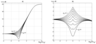Figure 1

In Figure 1a LAFC of a sensitivity threshold of the magnetometer for various values of damping factor of a magnetic system of variometer $\beta_{\mathrm{nat}}$ from 0.2 to 1 and the fixed value of damping factor of the magnetometer $\beta_0=0.82$ are shown. Correction curves in Figure 1b shows that real LAFC of noise of a variometer with NF at $0.4 <\beta_{\mathrm{nat}} < 0.7$ can be replaced with the approximate LAFC with a margin error isn't exceeding 2 dB. Therefore, to receive low noise level at the magnetometer output, it is necessary to increase natural frequency of a variometer itself to values approximately equal to the demanded top boundary frequency of the magnetometer, and for decrease a noise level at a zero and low frequencies – to increase conversion coefficient of a variometer.

### 5. Dependence of the Noise of Magnetometer From the Construct of a Variometer

In relation to a vicalloy quartz variometers it was claimed that "... good quality of magnetic system of sensor has some limit, to surpass which it is impossible any "rational" design of the sensor", and for the best designs of sensors the natural frequency of a quartz variometer is equal to about 1 Hz at the scale value equal 1 nT to m/mm [Yanovsky, 1963].

With transition to new materials there is an opportunity to receive the big magnetic moment at the small linear sizes of a magnet and it is essential (to 16 times) increase $M/J_m$ relation. For example, magnets from samarium-cobalt possess the maximum anisotropy (their residual magnetic induction is ranging from 7 to 10 kG and coercive force – from 18 to 12 kE respectively) and are capable to keep residual magnetization of sufficient value even at very great values of the degaussing factor. It gives the chance to reduce the linear sizes of a magnet in the direction orthogonal to its rotation axes to reduce the moment of inertia of mobile part of a variometer, having kept at the former level its magnetic moment. Thus, at the scale value of variometers equal 4 nT m/mm $(W_1(0)=0.125 \times 10^6$ rad/T), the magnetic system of a variometer on a basis of vicalloy has the natural frequency about 1 Hz, samarium-cobalt – about 4 Hz.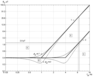Figure 2

Figure 2 shows the dependence of noise level upon the natural frequency for vicalloy based (line 1) and samarium-cobalt based (line 2) quartz variometers.

If to accept angular sensitivity of the optical-electronic converter $\theta_n=0.5 \times 10^{-7}$ rad, the threshold of sensitivity of the magnetometer at frequencies from 0 to own frequency of a variometer will be constant and equal $B_{\mathrm{nom}}=\theta_n/W_1(0) = 0.4$ pT (straight line at distance 0.4 pT from an axis of frequencies). At frequencies above own frequency of a variometer increasing the amplitude of noise (increase a threshold of sensitivity of the magnetometer) according to the quality of variometers (straight lines 1 or 2), with a speed of 6 dB/Oct is observed. These straight lines limit the areas characterizing distribution of noise on frequency at the output of magnetometers – areas 3 and 5. Reduction of conversion coefficient of variometers to $W_1(0) = 0.25 \times 10^5$ rad/T ("ruggedization" by 5 times), conducts to increase of frequency of own fluctuations of magnetic system from samarium-cobalt to 9 Hz, and to increase of a threshold of sensitivity of the magnetometer to 2 pT (dashed line).

Noise at its outputs in the low frequencies will increase at a size presented in Figure 2 by area 4. The variometer with magnetic system from samarium-cobalt (a straight line 2) in comparison with a variometer from vicalloy (a straight line 1) possesses significantly smaller noise level in the range of frequencies from 0 to 16 Hz (at a size of area 5). Noise of magnetometers at a frequency of 16 Hz makes 6.4 pT for samarium-cobalt, and 102.4 pT for vicalloy.

Extent of attenuation of own fluctuations of magnetic system of a variometer is of great importance for achievement of good noise characteristics of the magnetometer. From a ratio

\begin{eqnarray*} \omega_{\mathrm{df}} = \omega_{\mathrm{nat}} \sqrt{1- \beta_{\mathrm{nat}}^2} \end{eqnarray*}

follows that at change of dumping factor $\beta_{\mathrm{nat}}$ of a variometer from 0.3 to 0.7, natural frequency $\omega_{\mathrm{df}}$ of a variometer is ranging from 0.95 $\omega_{\mathrm{nat}}$ to 0.7 $\omega_{\mathrm{nat}}$. At further increase in dumping factor natural frequency of a variometer sharply decreases and at $\beta_{\mathrm{nat}} = 0.85$ it is already twice less than a reference value of frequency of own fluctuations. As a result the noise of the magnetometer at frequencies near the frequency of natural fluctuations of variometer magnetic system increases by size of area 6. On the other hand, deterioration of damping factor of magnetic system leads to increase of the noise connected with seismic impacts on a variometer and as optimum it is necessary to consider the value of dumping factor $\beta_{\mathrm{nat}}$ from 0.60 to 0.80. Frequency of own fluctuations of magnetic system of a variometer without NF, thus, makes from 0.6 to 0.8 $\omega_{\mathrm{nat}}$.

### 6. The Results of Studying of Quartz Magnetometers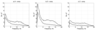Figure 3

The research of noise distribution at the outputs of quartz magnetometers have been executed by means of the analyzer FFT CF-300 of ONO SOKKI (Japan) in two frequency ranges – from 0.1 to 15 Hz and from 0.1 to 5 Hz, in natural electromagnetic field of Earth, in the conditions of lack of geomagnetic disturbances. Figure 3 shows the obtained noise spectra on outputs of quartz sensors DAMS [Askerov, 1989] for the first frequency range (the top curve) and the second one (the lower curve).

As a result of the analysis the following peak-to-peak values were obtained as noise level at the output of the sensors:

• in the first frequency range: $Bx$ – 7 pT, $By$ – 11 pT, $Bz$ – 6 pT;
• in the second one: $Bx$ – 3.5 pT, $By$ – 4 pT, $Bz$ – 2 pT.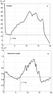Figure 4

In order to compare the noise levels of magnetometers with variometers made from different materials, the next Figure 4 shows the detailed spectra of signals at the output of the vicalloy based (a) and samarium-cobalt based (b) variometers in the frequency range from 1 to 20 Hz.

The noise amplitude begins to increase at lower frequencies (below 3 Hz) and the amplitude maximum reaches 40 pT for vicalloy variometers, while in the case of samarium-cobalt these values are about 7 Hz and 1.1 pT respectively.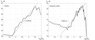Figure 5

The spectra of signals in logarithmic scale on Figure 5 let us to compare the experimental graphs of frequency distribution of noises at the outputs of the quartz magnetometers with curves characterizing the features of magnetic materials.

The slope of approximate straight lines equals 6 dB/Oct, which corresponds to model of noise distribution. This supports the conclusions drawn on the nature of noises at the output of quartz magnetometers.

### 7. Conclusions

The quality factor of magnetic system of quartz variometers depend on a form and the used magnetic materials. Samarium-cobalt has allowed to increase quality factor of magnetic system by 16 times. OEC of angular movements of magnetic system possess high coefficient of transformation and small dependence on factors of external environment and have angular sensitivity about $0.25 \times 10^{-7}$ rad. If the conversion factor of a variometer is taken as $0.125 \times 10^6$ rad/T, than the own frequency of the magnetometer with magnetic system from vicalloy is about 1 Hz, samarium-cobalt – 4 Hz, at a threshold of sensitivity 0.4 pT. These estimations are the limiting values of parameters of quartz variometers with OEC. They meet with a stock all the conditions of Intermaget for second data (1 pT on sensitivity and 0.2 Hz cut-off frequency) and give the chance to provide a network of magnetic observatories of Russia with magnetometers of a domestic production.

### References

Askerov, E. N., et al. (1989), CAIS digital automatic measuring station, Geophysical Equipment, issue 91, Mikhaylov G. N. (Ed.), p. 3–12, Nedra, Moscow.

Bobrov, V. N. (1961), The universal highly stable sensing device with a zero temperature coefficient for the magnetometers, variometers and microvariometers recording any component of a terrestrial magnetic field, Terrestrial Magnetism of II. Theoretical Bases of a Magnetometric Research Technique of Crust and Geomagnetic Measurements, IZMIRAN, issue 18(28), p. 55–67, IZMIRAN, Moscow.

Bryunelli, B. E., et al. (1959), Observations of short period variations of an electromagnetic field of the Earth, Izvestija Academy of Sciences of the USSR, Geophysics, no. 8, p. 1206–1213.

Hegymegi, L., L. Drimusz, Z. Koros (1986), Eotvos Institute DIMARS Quartz Magnetometer, Proc. of the Int. Workshop on Magnetic Observatory Instruments, Ottawa, Canada, 30 July–9 August 1986, p. 25–26, IWMOI, Ottawa.

Jankowski, J., et al. (1984), Long-Term Stability of a Torque-Balance Variometer with Photoelectric Converters in Observatory Practice, Geophysical Surveys, 6, no. 3–4, p. 367–380, doi:10.1007/BF01465552.

Klyuev, A. S. (1973), Automatic Control, 391 pp., Energy, Moscow.

Marianiuk, J. (1977), Photoelectric Converter for Recording the Geomagnetic Field Elements: Construction and Principles of Operation, 114 pp., Publ. Inst. Geophys. Pol. Acad. Sci., Poland.

Odintsov, V. I., V. E. Kolesnik (1982), Digital magnetovariation station CMVS-2, Geomagnetic Researches, p. 22–33, Radio and Communication, Moscow.

Rabinovich, S. G. (1972), Galvanometric Autocompensation Devices, 36–40 pp., Standards Publishing House, Moscow.

Yanovsky, B. M. (1963), Terrestrial Magnetism of II. Theoretical Bases of a Magnetometric Research Technique of Crust and Geomagnetic Measurements, 392–413 pp., Publishing house of the Leningrad University, Leningrad.

Received 28 September 2016; accepted 2 October 2016; published 18 October 2016.Citation: Odintsov V. I., V. G. Petrov (2016), Threshold sensitivity of quartz torsion magnetometers on a base of vicalloy and samarium-cobalt, Russ. J. Earth Sci., 16, ES5001, doi:10.2205/2016ES000577.

Generated from LaTeX source by ELXpaper, v.1.5 software package.